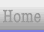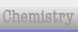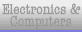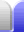# Fractions as Decimals & Percentages

Any fraction can be expressed as a decimal. Some are very simple to write, others are harder, since they may not ever end. The first digit after the decimal point is the tenths, the second digit after the decimal point is the hundredths, and so on.

110 = 0.1

1100 = 0.01

11100 = 0.11

377400 = 0.9425

Unfortuataly not all fractions have a multiple of 10 on the bottom line, but there are still many that can be easily written.

12 = 0.5

120 = 0.05

15 = 0.2

150 = 0.02

14 = 0.25

140 = 0.025

377400 = 0.9425

If a decimal repeats is it known as a recurring decimal. If the recurring decimal has only one digit that recurs the number can be written with a dot (or line) above the digit. Other numbers that have several numbers that recur can be written with a dot above the first and last digits in the group that recurs (or a line above the whole sequence/pattern).

13 = 0.333333...

 1⁄3 = 0. . 3

17 = 0.142857142857...

 1⁄7 = 0. . . 1 4285 7

What happens when we add three thirds?

13 = 0.333333...

3 x 13 = 33 = 1

3 x 0.333... = 0.999...

Does this means that 0.999999... ("zero point nine recurring") is the same as 1? (Answer on the Number Sets page.)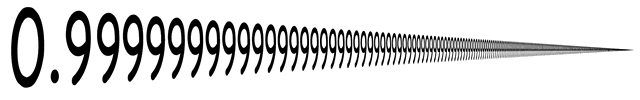### Handy fractions to remember

Some of the simple fractions pop up so often that it's very handy to remember the decimal and percentage equivalents.

Fraction Decimal Percentage
12 0.5 50 %
13 0.333... or 0.3 33⅓ %
14 0.25 25 %
15 0.2 20 %
16 0.1666... or 0.6 16 %
17 0.142857 1427 %
18 0.125 12.5 %
19 0.111... or 0.1 1119 %
110 0.1 10 %
111 0.0909... or 0.09 9111 %
112 0.08333... or 0.083 8 %
120 0.05 5 %
125 0.04 4 %
150 0.02 2 %
1100 0.01 1 %

Multiples of these fractions (ie, with numbers larger than 1 as the numerator) can simply be remembered or quickly worked out by adding smaller fractions together.

18 = 0.125 = 12.5 %

48 = 12 = 0.5 = 50 %

58 = 18 + 48 = 0.125 + 0.5 = 0.625 = 62.5 %

The sevenths fractions are a little curious because each one has the same digits in its repeating decimal sequence, but starts at a different digit in the sequence.

17 = 0.142857 = 1427 %

27 = 0.285714 = 2847 %

37 = 0.428571 = 4267 %

47 = 0.571428 = 5717 %

57 = 0.714285 = 7147 %

67 = 0.857142 = 8557 %

77 = 1 = 100 %Home Astronomy Chemistry Electronics & Computers Mathematics Physics Field Trips Turn on javascript for email link